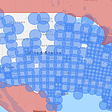Handwritten Signature Verification using Siamese Neural Network and One Shot Learning with Amazon Sagemaker

Handwritten Signature is one of the most popular and commonly accepted biometric hallmarks across industries like banks, insurance, forensic, etc., which is used to verify the different entities related to documents, forms, bank checks, etc., In case of standard classification, we first require a lot of different images (Imagine if you are doing this for a bank with thousands of customers). What if a new person joins or leaves the organization? You need to take the pain of collecting data again and re-train the entire model again. This is practically not possible especially for large organizations.

On the other hand, in a one shot classification, we require only one training example for each class. Hence it’s named as One Shot. You do not require too many instances of a class and only few are enough to build a good model. But the biggest advantage is that, let’s say in case of signature verification, the network will calculate the similarity for any new(unseen) signatures presented to it. Thus we say that network predicts the score in one shot.

In this blog post, we will look at how to leverage Amazon Sagemaker’s BYO script approach to train and deploy the Signature Verification model with One shot Learning using Siamese networks.

# Solution Overview

• One Shot Learning using Siamese Networks

• Implementation of FC Convolutional NN based on TensorFlow Keras

• Training Dataset — CEDAR Signature Database(http://www.cedar.buffalo.edu/NIJ/data/signatures.rar )

• BYO script approach with Amazon Sagemaker using Tensorflow container image

• Model deployment using Amazon Sagemaker Endpoint for real time Signature Verification

Convolutional NN Architecture — Siamese Network
Reference:
https://www.cs.cmu.edu/~rsalakhu/papers/oneshot1.pdf

# Few shot learning

The main challenge for a Deep Learning based classification model could be a larger dataset and the right tuning of hyperparameters for such a larger dataset.

But what if we need an automated system, which can successfully classify images to various classes given the data for each image class is quite less.

Few shot learning is such a problem. We can Few shot learning as a problem to classify data into K classes where each class has only few examples. The paper written by Gregory et. al, suggest ideas for building a Neural Network Architecture to solve this problem.

# Siamese networks

Siamese networks a Deep Neural Network architecture proposed by Gregory et. al in his paper Siamese Neural Networks for One-shot Image Recognition, the paper proposes an architecture where using Convolutional Nueral Networks one can tackle the problem of One Shot Learning.

The model aims to solve the basic problem of image verification, given that we have very few samples of image of each class or category.

The models aims to learn the embeddings of 2 separate images fed into the Neural Network, the two embeddings are used to calculate the L1 distance between the 2 embeddings. Once the distance embedding metric is calculated, the embedding is fed into a sigmoid unit which by the magic of back propogation, learns the correct set of hyperparameters to carry out the image verification.

# Amazon Sagemaker Architecture

I’ve used BYO Script approach with Amazon Sagemaker with Tensorflow 2.1 container image from Amazon ECR.

Refer to my github repo https://github.com/chakravn/signature-verification for the reference notebooks and scripts

Following details on files and directory in the above repo.

1. test — Directory contains test signature images

2. Data_prep_v2.ipynb — Data Preparation notebook

3. Signature_Verification_Sagemaker.ipynb — Notebook for Training and Inference

4. train.py — Training script

Please refer to “train.py” for the Siamese NN construct, Hyperparameters and model training using TF Keras.

You may need to start with the “Data_prep_v2.ipynb” notebook which covers downloading of CEDAR dataset, pre-processing of images using OpenCV followed by converting them into a pkl file for training in Amazon S3 bucket.

Following is the sequence of “Signature_Verification_Sagemaker.ipynb” on the training and inference for Handwritten Signature verification.

!pip install -U sagemaker

Import all of the required modules along with Sagemaker session, IAM role for Sagemaker execution and AWS region

`import osimport sagemakerfrom sagemaker import get_execution_roleimport tensorflow as tfsagemaker_session = sagemaker.Session()role = get_execution_role()region = sagemaker_session.boto_session.region_name`

If you want to test the training locally(on the same notebook instance only for debugging), please proceed with the following steps, which will help you on easy debug with lesser time on small amount of input data as the recommended Notebook instance usage is only as Data Scientist’s IDE not intend to run any heavy computation tasks like data processing, Training or Inference.

The setup.sh script will ensure that the pre-requisites are met in terms of root access or setting up the docker setup locally.

## !/bin/bash ./setup.sh

Run the estimator as below with “train_instance_type” as “local” which will run the docker image locally and initiate training.

`from sagemaker.tensorflow import TensorFlowdata_dir = ‘<local_path>’TF_estimator_local = TensorFlow(entry_point=’train.py’, role=role,train_instance_count=1, train_instance_type=’local’,framework_version=’2.1.0', py_version=’py3', script_mode=True )TF_estimator_local.fit({"train": f'file://{data_dir}' ,})`

# Training on Amazon Sagemaker

Copy the processed pkl file to Amazon S3 bucket

`pklfile = '../data/model_train/model_data_pre_processed.pkl'!aws s3 cp {pklfile} s3://<bucket_name>/<prefix>`

## Initiate Amazon Sagemaker Training

Define the S3 location of the directory holding the pkl file as “train_dir” and the S3 output location for the model. Then call the TensoFlow estimator with the entry_point as “train.py” which is available locally from the Notebook instance followed by “train_instance_count”, “train_instance_type”, “framework_version”(please refer to the doc on the supported TF versions), “py_version” and “script_mode” as True.

`from sagemaker.tensorflow import TensorFlowdir1 = 's3://<bucket>/<prefix>/'train_dir = dir1+"<model_dir_in_S3>"output_dir = dir1+"<output_dir_in_S3>""TF_estimator = TensorFlow(entry_point='train.py',role=role, train_instance_count=1, train_instance_type='ml.p2.xlarge',framework_version='2.1.0', py_version='py3', script_mode=True,output_path=output_dir)TF_estimator.fit({"train": train_dir})`

You can check the CloudWatch logs, utilization of the training instance and algorithm metrics in

AWS management console →Amazon Sagemaker →Training →Training Jobs

# Deploy Endpoint

Deploy the Amazon Sagemaker Endpoint for low latency real time Signature Verification. Optionally, you can configure Auto Scaling on endpoints as well. Please refer to https://docs.aws.amazon.com/sagemaker/latest/dg/endpoint-auto-scaling.html

# Inference — Signature Verification

Create the following functions to pre-process the signature images similar to what’s been done during the data preparation stage.

Note: Set the Threshold for the validation in the line “pred_class = pred_score >= 0.95” between 0 and 1. In this example I’ve set anything below or equal to 0.95 is considered to be “No Match”.

`import cv2import matplotlib.pyplot as pltdef morph(inp):    image = cv2.imread(inp)    result = image.copy()    image = cv2.cvtColor(image, cv2.COLOR_BGR2GRAY)    kernel = cv2.getStructuringElement(cv2.MORPH_RECT, (3,3))    opening = cv2.morphologyEx(image, cv2.MORPH_OPEN, kernel, iterations=1)    close = cv2.morphologyEx(opening, cv2.MORPH_CLOSE, kernel, iterations=2)    cnts = cv2.findContours(close, cv2.RETR_EXTERNAL, cv2.CHAIN_APPROX_SIMPLE)    cnts = cnts if len(cnts) == 2 else cnts    boxes = []    for c in cnts:        (x, y, w, h) = cv2.boundingRect(c)        boxes.append([x,y, x+w,y+h])    boxes = np.asarray(boxes)    left = np.min(boxes[:,0])    top = np.min(boxes[:,1])    right = np.max(boxes[:,2])    bottom = np.max(boxes[:,3])    result[close==0] = (255,255,255)    ROI = result[top:bottom, left:right].copy()    ROI = cv2.cvtColor(ROI, cv2.COLOR_BGR2GRAY)    retval, thresh_crop = cv2.threshold(ROI, 150, 255, cv2.THRESH_BINARY | cv2.THRESH_OTSU)    fin = cv2.resize(thresh_crop, (128, 64))    return findef match_sign(img1, img2):    x = np.array([np.array([        morph(img1),        morph(img2),     ])])    y_pred_1 = x[:, 0].reshape(len(x[:, 0]), 64, 128, 1)    y_pred_2 = x[:, 1].reshape(len(x[:, 1]), 64, 128, 1)    y_pred1 = y_pred_1.tolist()    y_pred2 = y_pred_2.tolist()    inputs = {"instances": [{"input_1": y_pred1, "input_2": y_pred2}] }        ##Prediction with endpoint    pred = predictor.predict(inputs)    pred_score = pred['predictions']    pred_class = pred_score >= 0.95    print("Predicted: {} => {}".format(pred_score, pred_class))        ##PLot    fig, ax = plt.subplots(1, 2, figsize=(20, 3))    ax.imshow(cv2.imread(img1))    ax.imshow(cv2.imread(img2))    ax.set_title("Signature-1")    ax.set_title("Signature-2")    plt.show()    plt.close()`

Now provide two different Signature images for the inference and results as below.

##Test1

img1 = ‘./test/b1.png’

img2 = ‘./test/b2.png’

match_sign(img1, img2)

`Predicted: 0.539490283 => False`
`##Test2img1 = './test/a1.png'img2 = './test/a2.png'match_sign(img1, img2)Predicted: 0.811340928 => False`
`##Test3img1 = './test/original_1_1.png'img2 = './test/original_1_18.png'match_sign(img1, img2)Predicted: 0.999214649 => True`

# Conclusion:

This is only a reference notebook and still there are lot of scope for further optimization. Image pre-processing plays a crucial part as the background and the pen color used for the handwritten Signature may vary from time to time on the handwritten Signatures.

References:

https://www.cs.cmu.edu/~rsalakhu/papers/oneshot1.pdf

--

--

--

## More from Chakravarthy Nagarajan

Principal AI/ML Specialist Solutions Architect at Amazon Internet Services Pvt Ltd

Love podcasts or audiobooks? Learn on the go with our new app.

## Breast Cancer classification using machine learning## Faster A.I. For Fortnite To PUBG Graphics Conversion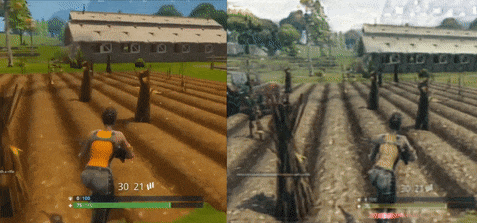## Machine learning: Types (Part 2)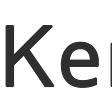## Practical-1 |Practical-2 | Practical-3 | Practical-4 | Practical-5 | Practical-6 | Practical-7 |…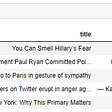## Avoid Reinforcement Bias When Fishing in the Same Pond## The impact of Machine Learning on the E̸v̸i̸d̸e̸n̸c̸e̸ Security Industry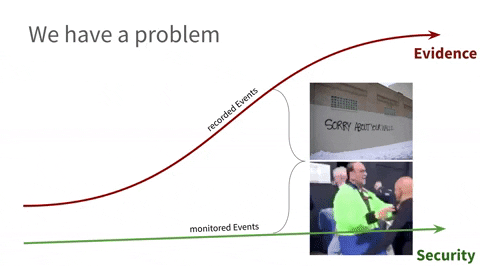## Scalable Gaussian Processes : An Introduction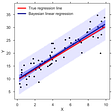## Chakravarthy Nagarajan

Principal AI/ML Specialist Solutions Architect at Amazon Internet Services Pvt Ltd

## Step 2: Preparing for a Technical interview — A Step by Step Guide: Create Job Portal profile